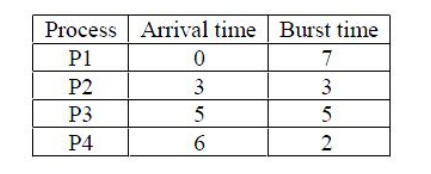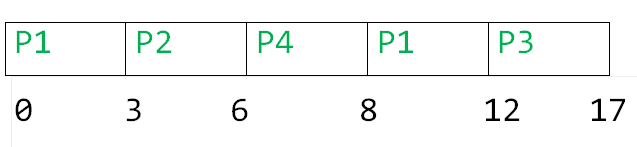Skip to content
Related Articles
GATE | GATE-CS-2017 (Set 1) | Question 16
• Last Updated : 15 Feb, 2018

Consider the following CPU processes with arrival times (in milliseconds) and length of CPU bursts (in milliseconds) as given below:If the pre-emptive shortest remaining time first scheduling algorithm is used to schedule the processes, then the average waiting time across all processes is _______ milliseconds.

Note: This questions appeared as Numerical Answer Type.
(A) 1
(B) 2
(C) 3
(D) 4

Answer: (C)

Explanation:Turn Around Time

```P1  = 12-0 = 12
P2 = 6-3 = 3
P3 = 17-5 = 12
P4 = 8 - 6 = 2
```

Waiting Time

```P1  = 12-7 = 5
P2 = 3-3 = 0
P3 = 12-5 = 7
P4 = 2 - 2 = 0
```

Average Waiting time = (7+0+5+0)/4 = 3.0

Therefore, option C is correct

Alternate Solution

Given, with arrival time and burst time:Using (preemptive) shortest remaining time first algorithm, gantt chart is:Therefore,
Average waiting time = ( 5 + 0 + 7 + 0 ) / 4 = 12 / 4 = 3

This explanation has been contributed by Mithlesh Upadhyay.

Attention reader! Don’t stop learning now. Learn all GATE CS concepts with Free Live Classes on our youtube channel.

My Personal Notes arrow_drop_up